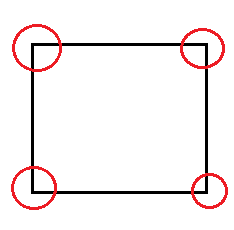# Problem: Sketch the atomic packing of the (100) plane in the FCC crystal structure. a) Determine the planar density for this plane and compare to the planar density for the (200) plane in FCC (i.e. sketch the (200) plane and determine its planar density). b) Based on your findings, are these planes crystallographically equivalent?

###### FREE Expert Solution

(1,0,0) plane for FCC structure:number of atoms = (1)+ (1/4) (4) = 2

(2a)1/2 = 4γ

a = 2 (2γ)1/2

Area = a2 = (2(2γ)1/2)2 = 8γ2

(2,0,0) plane for FCC structure:

100% (237 ratings)###### Problem Details

Sketch the atomic packing of the (100) plane in the FCC crystal structure.

a) Determine the planar density for this plane and compare to the planar density for the (200) plane in FCC (i.e. sketch the (200) plane and determine its planar density).

b) Based on your findings, are these planes crystallographically equivalent?